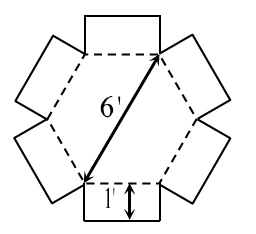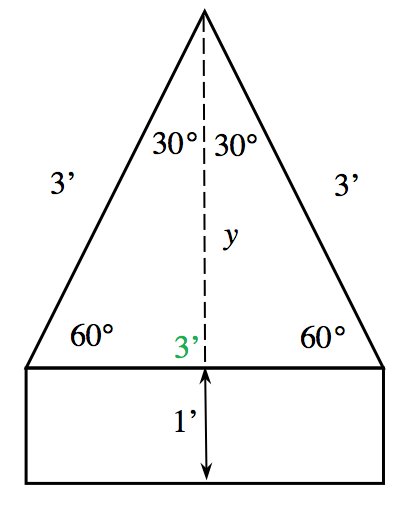### Home > INT2 > Chapter 9 > Lesson 9.1.4 > Problem9-48

9-48.Mr. Singer has a dining table in the shape of a regular hexagon. While he loves this design, he has trouble finding tablecloths to cover it. So he has decided to make his own tablecloth!

In order for his tablecloth to drape over each edge, he will add a rectangular piece along each side of the regular hexagon as shown in the diagram at right. Using the dimensions given in the diagram, what is the total area of fabric that Mr. Singer will need? (You do not need to consider the area needed for seams.)

Remember that a regular hexagon is made up of six equilateral triangles. Calculate the area of one of the interior triangles and one of the exterior rectangles and then multiply by $6$.

Refer to the diagram below.
The base of the triangle is $3'$ because the sides of an equilateral triangle are all equal.
Therefore, the area of the rectangle is $(3')(1') = 3$ ft$^2$.Determine the height ($y$) by using a $30^\circ-60^\circ-90^\circ$ triangle pattern. Since the hypotenuse of the formed right triangle is $3'$ and the base is $1.5'$, the height must be $1.5√ 3 '$.

The area of one equilateral triangle is $0.5(1.5√ 3 ')(3') ≈ 3.9$ ft$^2$.

Add the areas of the rectangle and triangle and then multiply by $6$.
$6(3.9' + 3')$

Total area $≈ 41.1$ ft$^2$